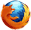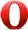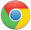# JavaScript sin() 方法JavaScript Math 对象

## 实例

Math.sin(3);

0.1411200080598672

## 浏览器支持Math.sin(x)

## 参数值

x 必需。一个以弧度表示的角。将角度乘以 0.017453293 （2PI/360）即可转换为弧度。

## 返回值

Number 参数 x 的正弦值。返回值在 -1.0 到 1.0 之间。

## 技术细节

 JavaScript 版本： 1

## 实例

var a=Math.sin(3);
var b=Math.sin(-3);
var c=Math.sin(0);
var d=Math.sin(Math.PI);
var e=Math.sin(Math.PI/2);

a,b,c,d, 和 e 输出结果:

0.1411200080598672
-0.1411200080598672
0
1.2246063538223772e-16
1JavaScript Math 对象